قانون سنل Snell\'s Law

# Snell's Law

Refraction is the bending of the path of a light wave as it passes across the boundary separating two media. Refraction is caused by the change in speed experienced by a wave when it changes medium., focused on the topics of "What causes refraction?" and "Which direction does light refract?" In that lesson, we learned that light can either refract (when slowing down while crossing the boundary) or away from the normal (when speeding up while crossing the boundary). The focus of Lesson 2 is upon the question of "By how much does light refract when it crosses a boundary?" In the  we learned that a comparison of the angle of refraction to the angle of incidence provides a good measure of the refractive ability of any given boundary. The more that light refracts, the bigger the difference between these two angles. In this part of Lesson 2, we will learn about a mathematical equation relating these two angles and the indices of refraction of the two materials on each side of the boundary.

###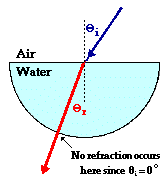A Lesson from the Laboratory

To begin, consider a hemi-cylindrical dish filled with water. Suppose that a laser beam is directed towards the flat side of the dish at the exact center of the dish. The angle of incidence can be measured at the point of incidence. This ray will refract, bending towards the normal (since the light is passing from a medium in which it travels fast into one in which it travels slow - FST). Once the light ray enters the water, it travels in a straight line until it reaches the second boundary. At the second boundary, the light ray is approaching along the normal to the curved surface (this stems from the geometry of circles). The ray does not refract upon exiting since the angle of incidence is 0-degrees (recall the If I Were An Archer Fishpage). The ray of laser light therefore exits at the same angle as the refracted ray of light made at the first boundary. These two angles can be measured and recorded. The angle of incidence of the laser beam can be changed to 5-degrees and new measurements can be made and recorded. This process can be repeated until a complete data set of accurate values has been collected. The data below show a representative set of data for such an experiment.

 Angle of Incidence (degrees) Angle of Refraction (degrees) 0.00 0.00 5.00 3.8 10.0 7.5 15.0 11.2 20.0 14.9 25.0 18.5 30.0 22.1 35.0 25.5 40.0 28.9 45.0 32.1 50.0 35.2 55.0 38.0 60.0 40.6 65.0 43.0 70.0 45.0 75.0 46.6 80.0 47.8 85.0 48.5

An inspection of the data above reveals that there is no clear linear relationship between the angle of incidence and the angle of refraction. For example, a doubling of the angle of incidence from 40 degrees to 80 degrees does not result in a doubling of the angle of refraction. Thus, a plot of this data would not yield a straight line. If however, the sine of the angle of incidence and the sine of the angle of refraction were plotted, the plot would be a straight line, indicating a linear relationship between the sines of the important angles. If two quantities form a straight line on a graph, then a mathematical relationship can be written in y = m*x + bform. A plot of the sine of the angle of incidence vs. the sine of the angle of refraction is shown below.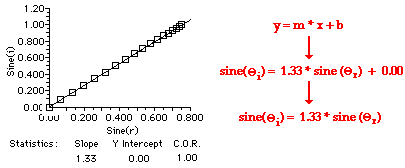The equation relating the angles of incidence (Θi) and the angle of refraction (Θr) for light passing from air into water is given as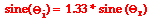Observe that the constant of proportionality in this equation is 1.33 -. Perhaps it's just a coincidence. But if the semi-cylindrical dish full of water was replaced by a semi-cylindrical disk of Plexiglas, the constant of proportionality would be 1.51 - the index of refraction value of Plexiglas. This is not just a coincidence. The same pattern would result for light traveling from air into any material. Experimentally, it is found that for a ray of light traveling from air into some material, the following equation can be written.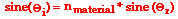where nmaterial = index of refraction of the material

This study of the refraction of light as it crosses from one material into a second material yields a general relationship between the sines of the angle of incidence and the angle of refraction. This general relationship is expressed by the following equation: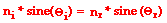where Θi ("theta i") = angle of incidence

Θr ("theta r") = angle of refraction

ni = index of refraction of the incident medium

nr = index of refraction of the refractive medium

This relationship between the angles of incidence and refraction and the indices of refraction of the two media is known asSnell's Law. Snell's law applies to the refraction of light in any situation, regardless of what the two media are.

### Using Snell's Law to Predict An Angle Value

As with any equation in physics, the Snell's Law equation is valued for its predictive ability. If any three of the four variables in the equation are known, the fourth variable can be predicted if appropriate problem-solving skills are employed. This is illustrated in the two examples below.

 Example ProblemsIn the following two examples, use Snell's law, the sine button on your calculator, a protractor, and the index of refraction values to complete the following diagrams. Measure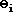, calculate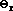, and draw in the refracted ray with the calculated angle of refraction.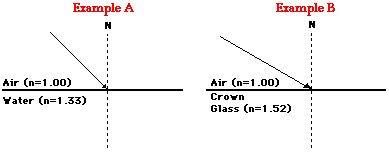In each of these two example problems, the angle of refraction is the variable to be determined. The indices of refraction (ni and nr) are given and the angle of incidence can be measured. With three of the four variables known, substitution into Snell's law followed by algebraic manipulation will lead to the answer.

Solution to Example A

First, use a protractor to measure the angle of incidence. An appropriate measurement would be some angle close to 45-degrees.

Second, list all known values and the unknown value for which you wish to solve:

 Given: ni = 1.00nr= 1.33Θi = 45 degrees Find: Θr = ???

Third, list the relevant equation:Fourth, substitute known values into the equation and algebraically manipulate the equation in order to solve for the unknown variable - Θr.

1.00 * sine (45 degrees) = 1.33 * الsine (Θr)

0.7071 = 1.33 * sine (Θr)

0.532 = sine (Θr)

sine-1 (0.532) = sine-1 ( sine Θr)

32.1 degrees = Θr

المصدر

### فيديو

«الرجوع للقسم»
تصميم وبرمجة المتحدة لخدمات الانترنت

جميع الحقوق محفوظة © لموقع لغة الروح